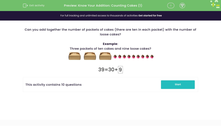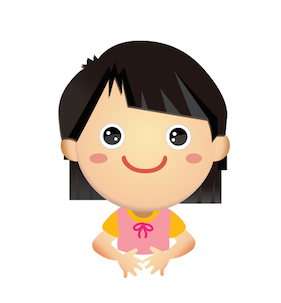# Partition Numbers into Tens and Ones Using Pictures.

In this worksheet, students will partition numbers into tens and ones.Key stage:  KS 1

Curriculum topic:   Number: Addition and Subtraction

Curriculum subtopic:   Solve Add/Subtract Problems to 100

Popular topics:   Counting worksheets

Difficulty level:#### Worksheet Overview

This activity is about splitting numbers into tens and ones.

For example, the number 42 is made up of 4 tens and 2 ones. So, 42 = 40 + 2

In these questions, we have loads of cakes - yummy!There are packets of cakes that contain 10 cakes:and also loose cakes that are not in the packets.We're going to add the cakes together.

Can you add together the number of packets of cakes (there are ten in each packet) with the number of loose cakes?

Example 1

Three packets of ten cakes and nine loose cakes?39 = 30 + 9

Did you see how we got that answer? There were 3 packets of cakes, so that means 3 groups of 10, which is 30. There were also 9 loose cakes.

Example 2

Can you add these cakes together?This time, there were 2 packets of cakes, so 2 groups of 10. There were also 5 loose cakes.

25 = 20 + 5

Are you ready to have a go at some questions now?### What is EdPlace?

We're your National Curriculum aligned online education content provider helping each child succeed in English, maths and science from year 1 to GCSE. With an EdPlace account you’ll be able to track and measure progress, helping each child achieve their best. We build confidence and attainment by personalising each child’s learning at a level that suits them.

Get started